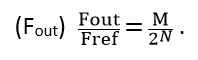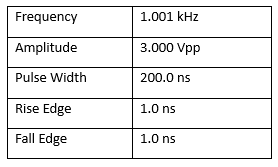EasyPulse Technology and Its Benefits

July 13, 2020

INTRODUCTION:

The majority of modern arbitrary/function waveform generators utilize DDS technology (Direct Digital Synthesis), but there are a few obvious defects using this technology directly. To solve these disadvantages, SIGLENT invented a pulse generating algorithm called EasyPulse technology. In this note, we will describe the basics of DDS and how EasyPulse can help get the best performance possible.

Direct digital synthesis (DDS) is a technique to produce an analog waveform (square, triangular, or sinusoidal) by generating a digital time-varying signal in digital form and then performing a digital-to-analog conversion. A basic Direct Digital Synthesizer consists of a frequency reference (often a crystal or oscillator), a numerically controlled oscillator (NCO) and a digital-to-analog converter (DAC), the block diagram is shown in Figure 1.

The reference clock is a fixed frequency (Fref). DDS generates the waveform by looking up the pre-loaded 2N data samples from memory.

The Tuning word (M) stored in the register determines the frequency of the output:Since the edges of square/pulse signals output through the DDS technology are fixed, and the duty-cycle is limited by the data length, there will be poor duty-cycle setpoint resolution at higher output frequencies.

When generating a square/pulse, the reference frequency should be an exact integral multiple of the output frequency. If it is not an exact integral multiple, it will introduce a deterministic jitter equal to one reference clock period.

When the Tuning word ＞1  (i.e. Fout ＞Fref /2N ), some points in the sample lookup table are skipped. This is generally not a big problem for sinusoidal waveforms, but for arbitrary waveforms with some important details (e.g. spikes), it may mean the loss of information.

2.EasyPulse technology and its benefits

To solve these problems, Siglent invented a pulse generating algorithm called EasyPulse, which is applied to all of the SDG X series waveform generators.

Based on this new technology, the SDG series waveform generator is capable of generating a pulse signal with low jitter, rapid rising and falling edge (independent from frequency), small duty cycle( pulse width), edge and pulse width can be adjusted in a wide range. Figure 3 is the block diagram of EasyPulse:

Take the SDG6052X series Pulse/Arbitrary Waveform Generator as an example, its specification for a pulse signal are as follows:

Equipped with the EasyPulse technology, the SDS6052X series Pulse/Arbitrary Waveform Generator delivers great performance. Let’s take a look at some real-world examples:

First and foremost, the EasyPulse technology could overcomes the additional jitter in Square/Pulse waveform generated by traditional DDS. To evaluate this excellent feature, we compared a DDS waveform generator with the SDG6052X with EasyPulse. In figure 4, we used a 12-bit scope to observe the differences. When the Pulse is trigged, measuring the next rise edge, we observe a 0.84 ns (which is equal to the period of the DDS clock, 1.2 GHz) peak-peak jitter if the pulse is generated by traditional DDS, while the jitter downs to 11.2 ps rms when we choose EasyPulse technology to generate the pulse with same configuration.

Another advantage of the EasyPulse technology is its ability to output the pulse with a small pulse width around 3.3 ns, even at very low frequency. For a 1 Hz pulse generated by DDS technology, there are 32768 points in one waveform length. If we built the pulse with only one sample point at the high level, we could get a pulse with the minimum pulse width (minimum duty ratio as well), which is about 30 ms (1 s/32768 ≈ 30.5 ms), as shown in figure 6. With EasyPulse, the width can be adjusted down to 3.3 ns as shown in figure 7.

In addition, with the EasyPulse technology, both the edge and pulse width can be adjusted over a wide range. The pulse width can be fine-tuned to the minimum of 3.3 ns with an adjustment step as small as 100 ps, at any frequency. In figures 8 and 9, we used a 12-bit scope with infinite persistence to show the rising edge changes with pulse width settings from 3.4 ns to 200 ns with 100 ps each step.